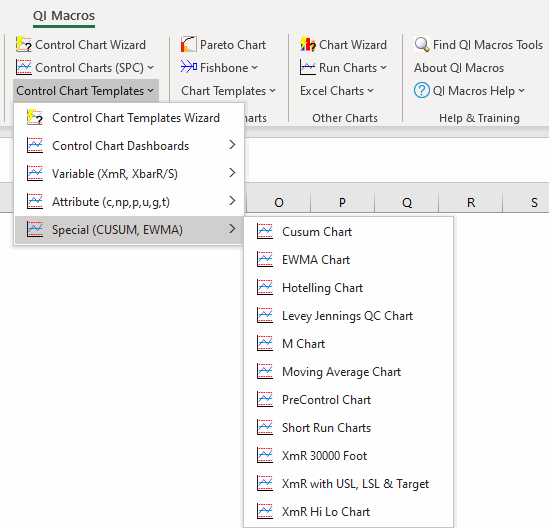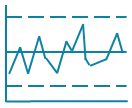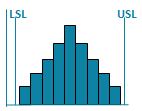# Moving Average Chart Template in Excel

## Use a Moving Average and Range chart when you have one sample and want a moving average.

### QI Macros Moving Average Template Has Three Options:

1. Moving Average Chart

• Input your data in column B
• Indicate the # of data points you want included in the moving average in cell K2 (Moving Average Period).
• Target (centerline) and standard deviation are calculated from your data but can be over written.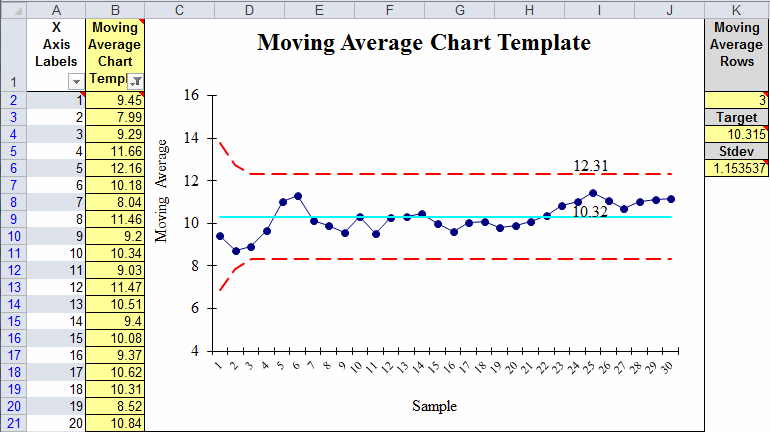2. Moving Average Rolling

Input your data in column B, the # of points used to calculate the moving average in cell M2 and the # of the most recent data points you want on the chart in cell M8.3. Wheeler Moving Average and Range

• Input your data in column B
• Indicate the # of data points you want included in the moving average in cell AA2 (Moving Average Period).
• This template uses different calculations for the Moving Average chart and includes a Range chart, Histogram with Cp Cpk, Capability Plot and Probability Plot.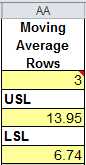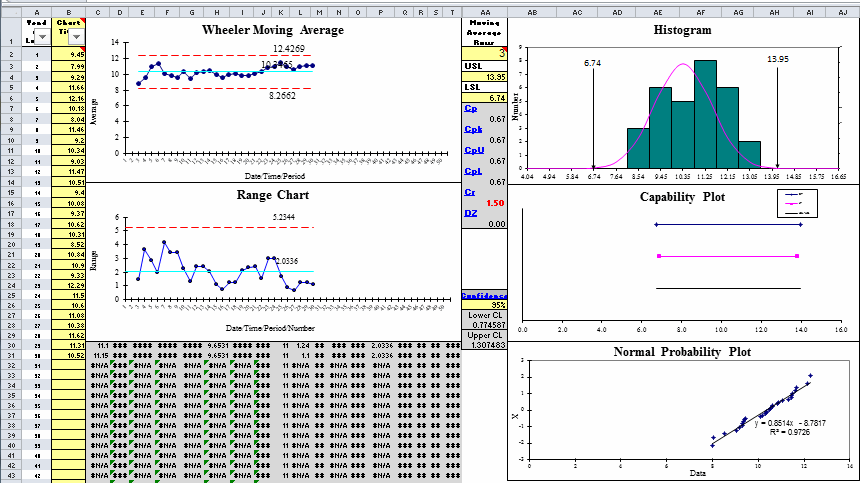### Moving Average Chart Template in QI Macros for Excel:

Find the Moving Average Chart template by clicking on the QI Macros menu > Control Chart Templates > Special (CUSUM, EWMA) > Moving Average Chart: